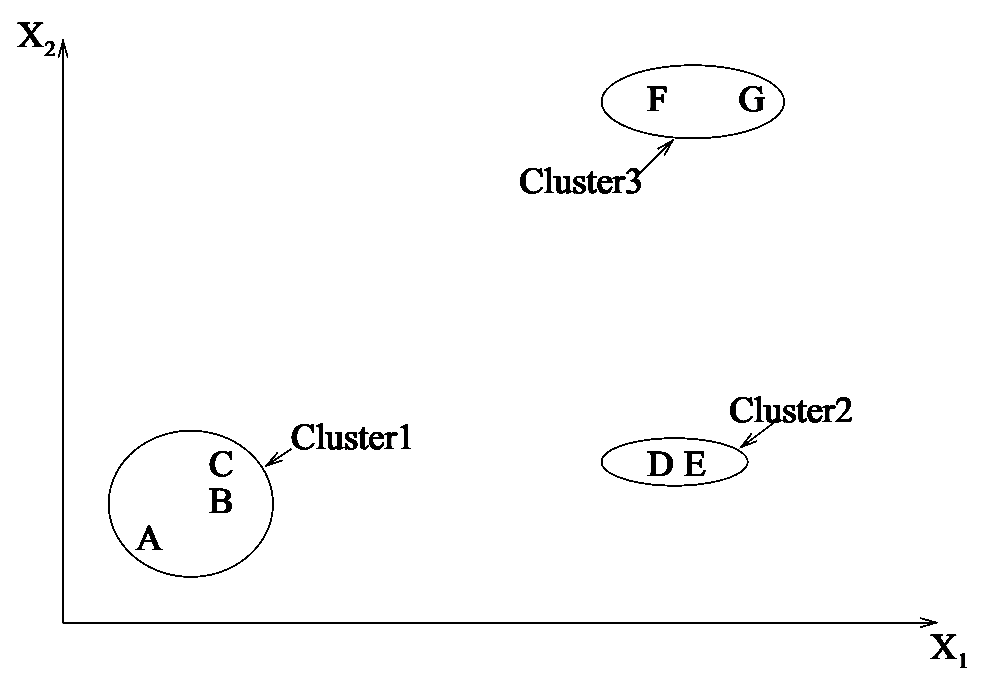# Hierarchical Clustering

Published on February 14, 2020 · 5 mins read

# Hierarchical Clustering

Hierarchical clustering is a method to group instances of a data set into a series of nested clusters or a tree of clusters called a dendrogram, which represents the similarity level between instances in the data set. An example hierarchical clustering is shown in Figures below. The figure shows a simple two-dimensional data set with three distinctive clusters. The data set is represented as in a hierarchical clustering model using a dendrogram. The dendrogram can be cut at any level (represented as a dotted horizontal line) to separate different patterns of the data set 1. The level of the cutoff line is subjective and may vary from one data set to another. Cutting a dendrogram from a higher level produces fewer patterns (clusters) 2.A simple data set with a possible dendrogram for hierarchical clustering algorithm. (a) Two dimensional data set with three obvious different groups. From JainA simple data set with a possible dendrogram for hierarchical clustering algorithm. (b) A dendrogram representation for a hierarchical clustering of the previous data set, From Jain

Based on the internal functioning of the hierarchical clustering algorithm, they can be divided into divisive and agglomerative types. The divisive method starts by assigning all instances into one cluster then partitions that cluster into two smaller clusters according to the similarities between instances. The process of sub-dividing each subcluster into another two clusters continues until each cluster contains single instance. In contrast, agglomerative hierarchical clustering starts by assigning each instance of the data set as a cluster, then starts to combine two most similar clusters into a single bigger cluster. This process is repeated recursively until a single cluster is achieved or a certain number of clusters are reached 3.

Whether divisive or agglomerative approach is used, a prerequisite to begin clustering is a proximity matrix, a symmetric matrix containing the similarity between every point in the data set using a distance function. This matrix is updated after each iteration to reflect the status of the data set under the method of clustering. The distance function can be Euclidean, Manhattan or any other distance function 3. Sections shows how time-based distance measures can be used to cluster temporal data sets.

To determine the similarity between clusters using proximity matrix in agglomerative method, one of the available linkage methods can be used 2:

• Single linkage: calculates the minimum distance between any items of two different clusters.
• Complete linkage: calculates the maximum distance between any items of two different clusters.
• Average linkage: calculates the average distance between all items of two different clusters.
• Centroid linkage: calculates the distance between centre of two different clusters.

Due to the time complexity hierarchical clustering can not be used with very large data sets which can not fit the memory. Moreover, the nature of the algorithm do not allow to reconsider the previous steps of the recursive clustering operation (dividing or joining) in contrast with the other clustering technique which we see before 2.

Reference

1. Jain, A. K., Murty, M. N. and Flynn, P. J. (1999) ‘Data clustering: a review’, ACM computing surveys (CSUR). ACM Press, 31(3), pp. 264–323. doi: 10.1145/331499.331504.

2. Wang, X. Y. (2006) Fuzzy Clustering in the Analysis of Fourier Transform Infrared Spectra for Cancer Diagnosis. The University of Nottingham.  2 3

3. Zaki, M. J. and Meira, M. J. (2014) Data Mining and Analysis: Fundamental Concepts and Algorithms, Cambridge University Press. New York: Cambridge University Press.  2

Polla Fattah